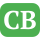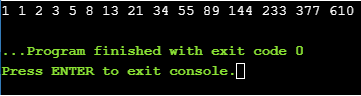CppBuzz.com

# C++ Program to Generate Fibonacci Series

```#include<iostream>
using namespace std;

#define NUMBER 15

int main()
{
int a, b;
a=b=1;

cout<<a<<" "<<b<<" ";

for(int i=0; i<NUMBER-2; i++){
int temp = a;
a = b;
b = temp + b;
cout<<b<<" ";
}

return 0;
}

```

## Generating Fibonacci Series of any number